# Plot for distribution of statistic and p-value

2018-04-18

To understand the concept of p value is very important. To teach the the distribution of common statistic( $$\chi^2$$ for chisq.test() , t for Student’s t-test , F for F-test) and concept of the p-value, plot.htest() function can be used.

## Homepage of this package

https://cardiomoon.github.io/webr/index.html

## Package Installation

You can install this package form the github. Currently, package webr is under construction and consists of only one function - plot.htest().

#install.packages("devtools")
devtools::install_github("cardiomoon/webr")

## Coverage of plot.htest()

The plot.htest() function is a S3 method for class “htest”. Currently, this function covers Welch Two Sample t-test, Pearson’s Chi-squared test, Two Sample t-test, One Sample t-test, Paired t-test and F test to compare two variances.

## For Chi-squared Test

You can show the distribution of chi-squre statistic and p-value.

 require(moonBook)
require(webr)

# chi-squared test
x=chisq.test(table(acs$sex,acs$DM))
x

Pearson's Chi-squared test with Yates' continuity correction

data:  table(acs$sex, acs$DM)
X-squared = 3.1296, df = 1, p-value = 0.07688
 plot(x)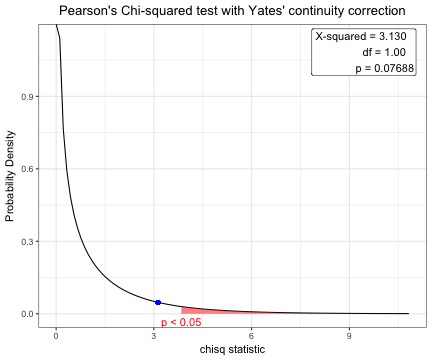## For one sample t-test

You can show the distribution of t-statistic and p-value in one sample t-test.

t.test(acs$age,mu=63)  One Sample t-test data: acs$age
t = 0.77978, df = 856, p-value = 0.4357
alternative hypothesis: true mean is not equal to 63
95 percent confidence interval:
62.52736 64.09574
sample estimates:
mean of x
63.31155 
plot(t.test(acs$age,mu=63))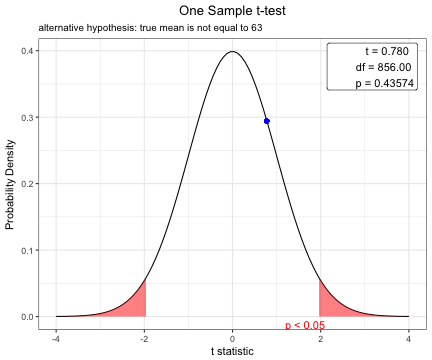## Student t-test to compare means for two independent samples Before performing a t-test, you have to compare two variances. ### F test to compare two variances x=var.test(age~DM,data=acs) x  F test to compare two variances data: age by DM F = 1.2383, num df = 552, denom df = 303, p-value = 0.0376 alternative hypothesis: true ratio of variances is not equal to 1 95 percent confidence interval: 1.012416 1.505776 sample estimates: ratio of variances 1.238288  plot(x)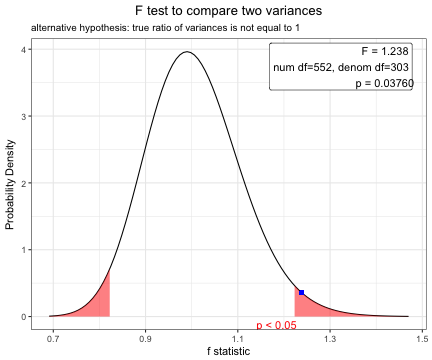### Use for Two Sample t-test for independence samples Based on the result of var.test(), you can perform t.test with default option(var.equal=FALSE). x=t.test(age~DM,data=acs) x  Welch Two Sample t-test data: age by DM t = 0.58982, df = 682.36, p-value = 0.5555 alternative hypothesis: true difference in means is not equal to 0 95 percent confidence interval: -1.112568 2.068014 sample estimates: mean in group No mean in group Yes 63.48101 63.00329  plot(x)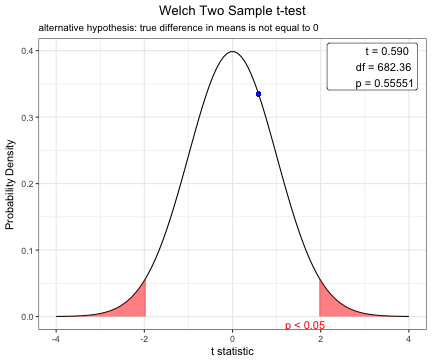## Student t-test using pooled variance To compare means of body-mass index between male and female patients, perform F test first. var.test(BMI~sex,data=acs)  F test to compare two variances data: BMI by sex F = 1.2078, num df = 254, denom df = 508, p-value = 0.07756 alternative hypothesis: true ratio of variances is not equal to 1 95 percent confidence interval: 0.9794315 1.5008098 sample estimates: ratio of variances 1.207759  plot(var.test(BMI~sex,data=acs))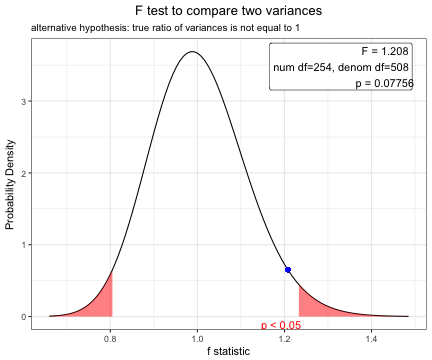Based on the result of F test, you can perform t-test using pooled variance. x=t.test(BMI~sex,data=acs,var.equal=TRUE) x  Two Sample t-test data: BMI by sex t = -0.50823, df = 762, p-value = 0.6114 alternative hypothesis: true difference in means is not equal to 0 95 percent confidence interval: -0.6348532 0.3737344 sample estimates: mean in group Female mean in group Male 24.19492 24.32548  plot(x)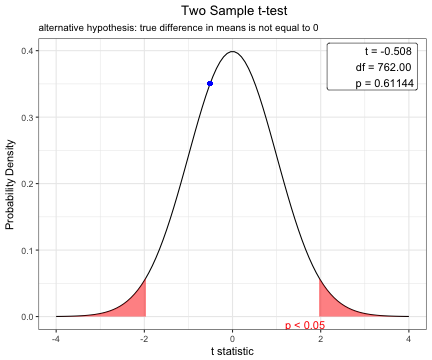## Paired t-test You can show the distribution of t-statistic and p-value in paired t-test. x=t.test(iris$Sepal.Width,iris\$Petal.Width,paired=TRUE)
plot(x)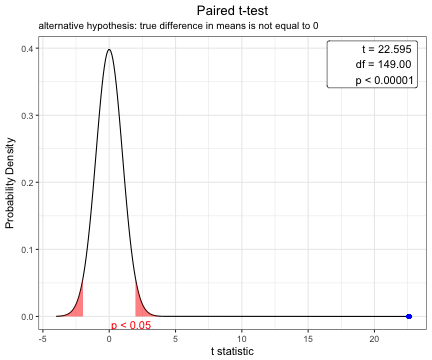## Options for t-test

You can change the options of t.test.

x=t.test(BMI~sex, data=acs,conf.level=0.99,alternative="greater",var.equal=TRUE)
plot(x)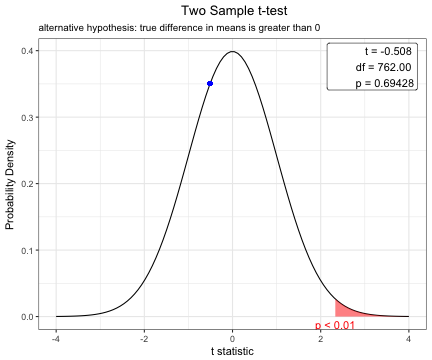## Vignette for PieDonut() function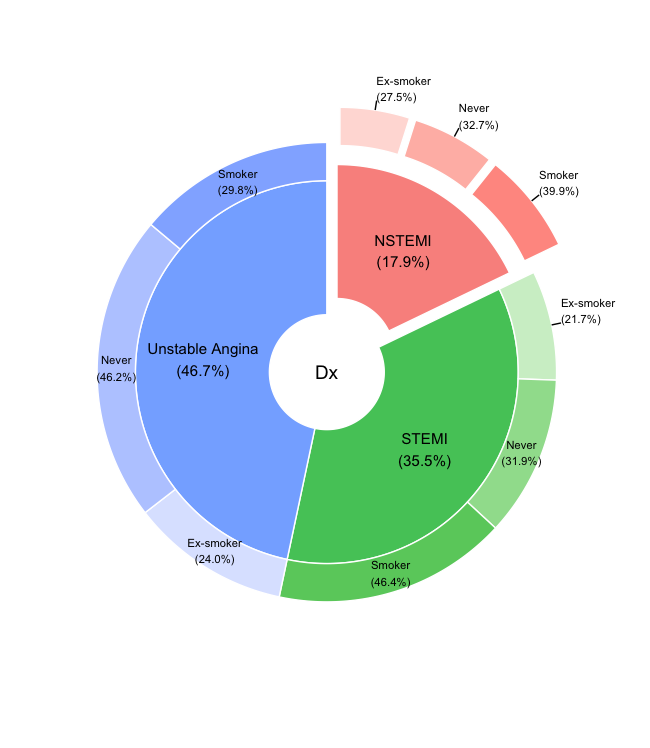http://rpubs.com/cardiomoon/398623